# Design and Analysis of the Speed and Torque Control of IM with DTC Based ANN Strategy for Electric Vehicle Application

Design and Analysis of the Speed and Torque Control of IM with DTC Based ANN Strategy for Electric Vehicle Application

Laboratory of L2GEGI, Faculty of Applied Sciences, University Ibn Khaldoun of Tiaret, BP 78 size Zaaroura 14000, Tiaret, Algeria

University Degli Studi of Cassino Via G. Di Biasio 43 I-03043 Cassino (FR), Department of Automation, Cassino, Italy

Corresponding Author Email:
arariarabah@gmail.com
Page:
181-188
|
DOI:
https://doi.org/10.18280/ti-ijes.632-410
18 February 2019
|
Accepted:
29 April 2019
|
Published:
30 June 2019
| Citation

OPEN ACCESS

Abstract:

The aim of this paper is the developments a strategy of control of an induction motor (IM) used as a propulsion system of an electric vehicle (EV). The proposed strategy at different operating conditions using a direct torque control (DTC) technique combined with an Artificial Neural Network (ANN) utilizes the stator flux as control variable and the flux level is selected in accordance with torque demand of the EV to achieve a high drive performance. Simulation results on a test vehicle propelled by two 38-kW induction motors showed that the proposed control approach operates satisfactorily. The analysis and simulations lead to the conclusion that the proposed system is feasible and can be tested on experimental bench.

Keywords:

artificial neural network control (ANNC), direct torque control (DTC), DC/DC converters, DC/AC inverter, electric vehicle (EV), induction motor (IM) drives

1. Introduction

Electric Vehicles (EVs) are a solution for the environmental problems caused by vehicles with internal combustion engines. The advantages of EVs include energy efficiency, virtually lack of pollution, and the availability of electric energy through electric distribution systems. Among disadvantages, they have low energy density and long charging time for the present batteries. Hence, optimal energy management is very important in EVs. The other major factors include optimum design of the motor, selection of a proper drive, and optimal control strategy.

As a primary power source for small aircrafts, the Fuel cells can be used. However, combining an electrical storage system with the fuel cell can lead to a better performance . Therefore, rechargeable batteries are used as a secondary source to boost the fuel cell power during high power demand.

For EVs propulsion, the cage induction motor seems to be candidate that better fulfills the major above-mentioned features [2, 3]. Induction motor drives control techniques are well treated in the literature. The most popular is the so-called vector control technique that is now used for high impact automotive applications (EV and Hybrid EV). In this case, the torque control is extended to transient states and allows better dynamic performances . Among these techniques, DTC appears to be very convenient for EV applications .

DTC has the advantage of not requiring speed or position encoders and uses voltage and current measurements only.

Flux, torque, and speed are estimated. It also has a faster dynamic response due to the absence of the PI current controller. The input of the motor controller is the reference speed, which is directly applied by the pedal of the vehicle. Furthermore, DTC typical advantages are not sufficient. EVs induction motor drive has also to possess a high efficiency in order to extend the running distance per battery charge. Indeed, EV motors have a high torque-to-volume ratio and a wide speed operation range. As a consequence, these motors are characterized by their low inductance and high current density, so that they run at high speed and produce a high starting torque. Due to the low inductance coil design, the current ripple caused by PWM switching makes a significant amount of eddy current losses and hysteresis losses, especially in high-speed operation. If we simply neglect the iron losses, then it detunes the overall vector controller and results in an error in the torque control .

The structure of the presented work is organized as follow: The description of the electric traction system and the physical modeling of different part of our system with their equations model are set in section 2. The control strategy applied to EV is set in section 3. The simulations results of the studied are presented in section 4. Section 5 summarizes the work done in the conclusion.

2. Electric Traction System

2.1 Electric vehicle analysis

This section derives the driving power to ensure vehicle operation (Figure 1) .

$F_w=F_{ro}+F_{sf}+F_{add}+F_{cr}$ (1)

The rolling resistance force F is produced by the tire flattening at the roadway contact surface.

$F_{ro}=\mu mg \cos{\alpha}$ (2)

μ is a non linearly dependent of the vehicle speed, type, tire pressure, and road surface characteristic. It increases with vehicle speed and also during vehicle turning manoeuvres.

## 1.png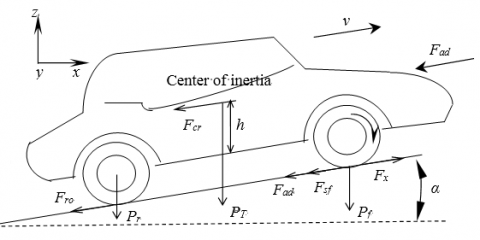Figure 1. Elementary forces acting on a vehicle

The rolling resistance force can be minimized by keeping the tires as much inflated as possible.

$F_{sf}=k_Av$ (3)

Aerodynamic drag, $F_{add}$ is the viscous resistance of air acting upon the vehicle.

${{F}_{add}}=\frac{1}{2}\xi {{C}_{w}}{{A}_{f}}{{(v+{{v}_{0}})}^{2}}$ (4)

The climbing resistance (

with positive operational sign) and the downgrade force (  with negative operational sign) is given by 

${{F}_{cr}}=\pm mg\sin \alpha$ (5)

The tractive force in an electric vehicle is supplied by the electric motor in overcoming the road load. The equation of motion is given by

${{k}_{m}}m\frac{dv}{dt}=F-{{F}_{w}}$ (6)

The net force ($F-F_w$), accelerates the vehicle (or decelerates when FW exceeds F).

The power required to drive a vehicle has to compensate the road load

$P_v=vF_w$ (7)

The mechanical equation (in the motor referential) used to describe each wheel drive is expressed by

$J\frac{d{{\omega }_{r}}}{dt}+{{T}_{B}}+{{T}_{L}}={{T}_{em}}$ (8)

The following equation is derived due to the use of a reduction gear.

${{\omega }_{wheel}}=\frac{{{\omega }_{m}}}{G}{{\omega }_{r}},{{T}_{wheel}}={{T}_{m}}G{{\eta }_{t}}$ (9)

The load torque in the motor referential is given by:

${{\omega }_{wheel}}=\frac{{{T}_{Lwheel}}}{G}{{\omega }_{r}}=\frac{r}{G}{{F}_{\omega }}$ (10)

The vehicle global inertia moment in the motor referential is given by:

$J={{J}_{W}}+{{J}_{V}},{{J}_{V}}=\frac{1}{2}m\frac{{{R}^{2}}}{{{i}^{2}}}(1-\lambda )$ （11）

If the adhesion coefficient of the road surface is high, then $\lambda$ is usually low and can be neglected.

## 2.pngFigure 2. Main components of electric vehicle

2.2 Battery and DC/DC converter

This part of the vehicle ensures the charging and discharging of the battery through a DC/DC buck boost converter from the fuel cell arriving at the DC bus .

The System Storage (SS) is connected to the DC voltage bus via a bi-directional Buck-Boost DC/DC converter, as shown in Figure 3. The SS will operate in charging, discharging or floating modes depending on the energy requirements and these modes are managed according to the DC bus voltage at the SS point of coupling. Consequently, the SS is required to provide necessary DC voltage level under different operating modes of the vehicle. When charging, switch S2 is activated and the converter works as a boost circuit; otherwise, when discharging, switch S1 is activated and the converter works as a buck circuit. When the voltage at the DC link is lower than the voltage reference, switch S1 is activated. Alternatively, when the voltage at the DC link is higher than the voltage reference, switch S2 is activated. The FC-battery system response to transient variations is characterized by an inherent time constant , In such cases, capacitors along the DC vehicle can act as virtual inertia to supply the shortfall or absorb the surplus of energy [8, 10]. The DC-link power balance can be expressed by the following differential equation:

$V_{dc}i_{dc}=P_{FC}+P_{bat}-P_{EV}$ (12)

Neglecting the losses in the power converters, battery, filtering inductors and transformer and also the harmonics due to switching actions, the power balance of the integrated hybrid distributed generation system (DGS) with energy storage is governed by:

$V_{dc}i_{dc}=CV_{dc}\frac{dV_{dc}}{dt}=P_{PV}+P_{W}+P_{bat}-P_{load}$ (13)

The objective of the battery converter is to maintain constant voltage at the DC link, so the ripple in the capacitor voltage is much lower than the steady-state voltage [11, 12].

## 3.png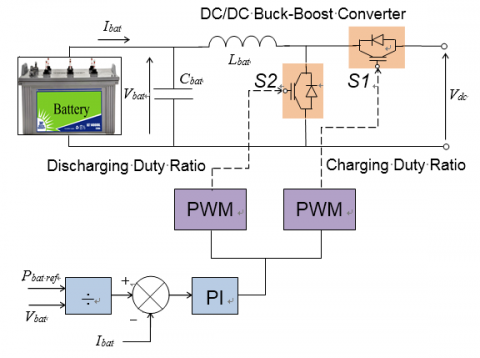Figure 3. DC/DC converter and battery control system

2.3 Induction motor model

The state space representation of the induction motor with the stator currents and the rotor flux linkages components as state variables can be written as [13, 14]:

$\left[ \begin{matrix} \frac{d}{dt}{{i}_{sd}} \\ \frac{d}{dt}{{i}_{sq}} \\ \frac{d}{dt}{{\psi }_{rd}} \\ \frac{d}{dt}{{\psi }_{rq}} \\\end{matrix} \right]=\left[ \begin{matrix} -\left( \frac{{{R}_{s}}}{\sigma {{L}_{s}}}+\frac{1-\sigma }{\sigma {{T}_{r}}} \right) & 0 & \frac{{{L}_{m}}}{\sigma {{L}_{s}}{{L}_{r}}{{T}_{r}}} & \left( \frac{{{L}_{m}}}{\sigma {{L}_{s}}{{L}_{r}}} \right){{\omega }_{r}} \\ 0 & -\left( \frac{{{R}_{s}}}{\sigma {{L}_{s}}}+\frac{1-\sigma }{\sigma {{T}_{r}}} \right) & -\left( \frac{{{L}_{m}}}{\sigma {{L}_{s}}{{L}_{r}}} \right){{\omega }_{r}} & \frac{{{L}_{m}}}{\sigma {{L}_{s}}{{L}_{r}}{{T}_{r}}} \\ \frac{{{L}_{m}}}{{{T}_{r}}} & 0 & \frac{-1}{{{T}_{r}}} & p{{\omega }_{r}} \\ 0 & \frac{{{L}_{m}}}{{{T}_{r}}} & -p{{\omega }_{r}} & \frac{-1}{{{T}_{r}}} \\\end{matrix} \right]\left[ \begin{matrix} {{i}_{sd}} \\ {{i}_{sq}} \\ {{\psi }_{rd}} \\ {{\psi }_{rq}} \\\end{matrix} \right]+\left[ \begin{matrix} \frac{1}{\sigma {{L}_{s}}} & 0 \\ 0 & \frac{1}{\sigma {{L}_{s}}} \\ 0 & 0 \\ 0 & 0 \\\end{matrix} \right]\left[ \begin{matrix} {{v}_{sd}} \\ {{v}_{sq}} \\\end{matrix} \right]$ (14)

where, Tr is the rotor time constant and σ is the leakage coefficient. The electromagnetic torque and the rotor speed are given by:

${{T}_{em}}=\frac{3}{2}p\frac{{{L}_{m}}}{J{{L}_{r}}}({{\psi }_{rd}}{{i}_{sq}}-{{\psi }_{rq}}{{i}_{sd}})$ (15)

$\frac{d{{\omega }_{r}}}{dt}=\frac{p}{J}{{T}_{em}}-\frac{B}{J}{{\omega }_{r}}-\frac{p}{J}{{T}_{l}}$  (16)

3. Control Strategy Applied to EV

3.1 Direct torque control (DTC)

DTC is a control philosophy exploiting the torque and flux producing capabilities of ac machines when fed by a voltage source inverter that does not require current regulator loops, still attaining similar performance to that obtained from a vector control drive.

3.2 Behavior of the stator flux

In the (d, q) reference, the stator flux can be obtained by the following equation:

${{\bar{V}}_{s}}={{R}_{s}}{{\bar{I}}_{s}}+\frac{d}{dt}{{\bar{\psi }}_{s}}$ (17)

3.3 Behavior of the torque

The electromagnetic torque is proportional to the vector product between the vector of stator and rotor flux according to the following expression 

${{T}_{em}}=k({{\bar{\psi }}_{s}}\otimes {{\bar{\psi }}_{r}})=k|{{\psi }_{s}}||{{\psi }_{r}}|\sin (\delta )$ (18)

with:

${{\bar{\psi }}_{s}}$ is the vector of stator flux;

${{\bar{\psi }}_{r}}$ is the vector of rotor flux;

$\delta$ is the angle between the vectors of stator and rotor flux.

3.4 Development of the commutation strategy

In order to exploit the operation possible sequences of the inverter on two levels, the classical selection table of the DTC is summarized in table 1.

Table 1. Selection table for direct torque control

 ∆ψs ∆Tem S1 S2 S3 S4 S5 S6 1 1 V2 V3 V4 V5 V6 V1 0 V7 V0 V7 V0 V7 V0 -1 V6 V1 V2 V3 V4 V5 0 1 V3 V4 V5 V6 V1 V2 0 V0 V7 V0 V7 V0 V7 -1 V5 V6 V1 V2 V3 V4

## 4.pngFigure 4. Partition of the complex plan in six angular sectors S I = 1…

It shows the commutation strategy suggested by Takahashi [14, 16], to control the stator flux and the electromagnetic torque of the induction motor. Figure 4 gives the partition of the complex plan in six angular sectors S I = 1… 6.

3.5 Proposed ANN speed controller

The neural network-based model can take various forms: it can be an artificial neural network (ANN) or a fuzzy neural network etc. [17-19], and there is also the possibility of using different typaes of speed tuning signals. It is believed that some of these solutions can give high accuracy and are relatively robust to parameter variations even at extremely low stator frequency.

The conventional PI speed controller was replaced by an Artificial Neural Network with the goal to get optimal performances for the closed loop control schema (Figure 5). This regulator has one input which is the error between the reference speed $\omega_r^*$ and process output speed [20, 21].

## 5.png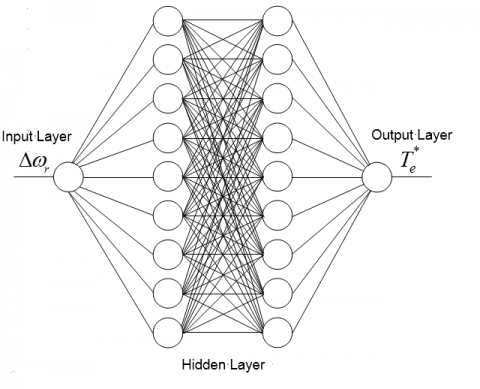Figure 5. Artificial neural network representation for speed controller

The output of ANN controller is applied to the entry of the process. After several tests, the architecture with two hidden layers was adopted [20, 22].

The basic DTC scheme for ac motor drives is show in Figure 6.

## 6.png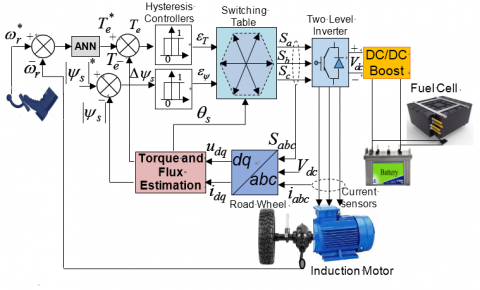Figure 6. Basic direct torque control for induction motor drives

4. Simulation Results and Discussion

Numerical simulations have been carried out, on an EV propelled by a 38 kW induction motor drive which ratings are summarized in the appendix (Table 2). The objectives of the carried-out simulations are to assess the efficiency and dynamic performances of the proposed control strategy (figure 7).

First, Figure 8 show the induction motor speed controlled with ANN in DTC. At the beginning of the simulation process, different speed levels are applied to the control of the induction motor until it reaches its equilibrium state.

Figure 9 shows the electromagnetic torque response and its zoom with PI and ANN, there are variations in the torque that corresponds to the variation of the reference speed at different times. Our order always intervenes to put the stable system.

Table 2. Induction motor parameters

 Components Rating values Rated power 38 kW Stator resistance Rs=0.01965 Ω Rotor resistance Rr=0.01909 Ω Stator/rotor inductance Ls=Lr=0.0397 H Mutual inductance Lm=1.354 H Moment of inertia J=0.09526 Kg.m2 Viscous friction f=0.05479 N.m/rad/sec Number of pole pairs p=2

## 7.png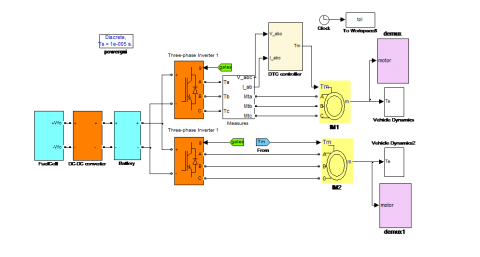Figure 7. The structure of the simulation model of the electric vehicle

## 8.png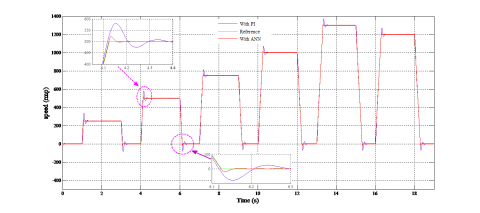Figure 8. Speed responses of EV

## 9.png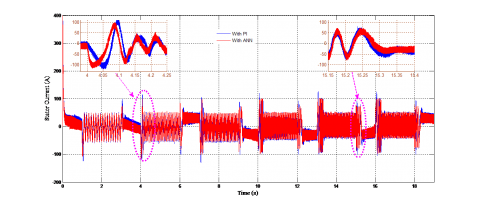Figure 9. Electromagnetic torque responses

## 10.png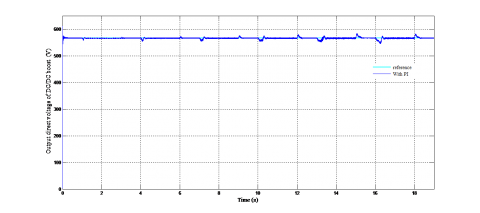Figure 10. DC boost output voltage

## 11.png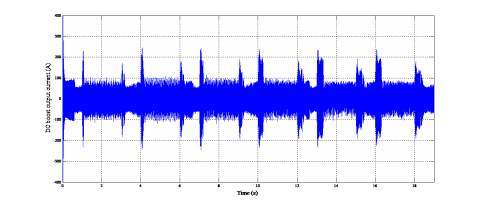Figure 11. DC boost output current

## 12.png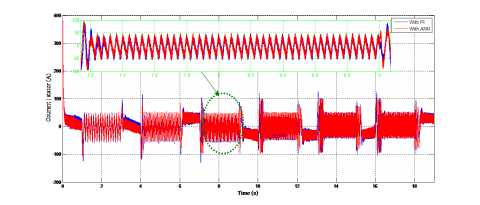Figure 12. Stator current of IM during speed change

## 13.pngFigure 13. Flux response of the proposed control

The results of the electric dynamics vehicle during starting and with change the acceleration pedal position and a varied road profile demonstrate that ANN based DTC reduces the current ripples and large torque compared with the DTC based PI controller.

Figures 10 and 11 shows the output current and voltage from the boost, these quantities ensure the power supply to the vehicle after the conversion of the DC bus through the inverter to supply the induction motor controlled by the ANNDTC.

Figures 12, illustrate stator current of IM, oscillations appear at each period corresponding to changes in vehicle speeds. The Figure 13 shows the stator flux, at the beginning of the operation the amplitude of the flux vector increases.

Then, the amplitude remains constant and equal to its reference value with a low ripple, thus revealing a correct regulation of the flux decoupled from that of the torque during the transient regimes.

The control strategy with the ANN let the dc bus voltage and the current always maintained constant with high performance compared with the PI controller.

From these results, it can be said that the training system meets the shifting requirements at different levels.

5. Conclusions

This work presented a detailed dynamic model of an EV that is associated with a DTC control use the ANN strategy for an induction motor drive.

The design method for Direct Torque Control of induction motor using Artificial Neural Network Controller replacing the PI regulator block have been successfully developed and implanted in MATLAB-Simulink environment. The performance of the speed motor electromagnetic torque behavior of the motor model using Neural Network Controller replacing both PI controller of Conventional DTC of IM have been evaluated and compared to use in electric vehicle drives. The results of simulation show that the proposed ANN for DTC provides better performance than that of conventional DTC technique use the PI regulator. The Proposed regulator based DTC significantly and easily tracks the reference speed and torques thereby improves the efficiency of speed-torque of induction motors with faster responses for fast changing of speed reference and torque as that of Electric Vehicles in any uneven roads conditions. The proposed ANN models are also simple and can be implemented easily with DSP or Dspace platform.

Nomenclature
 µ dynamic viscosity, kg. m-1.s-1 AC Alternating Current Af frontal surface area of the vehicle, m2 ANNC Artificial Neural Network Control Cw aerodynamic drag coefficient, d, q Synchronous reference frame index DC Direct Current DTC Direct Torque Control EVs Electric Vehicles Fadd Aerodynamic drag force FC Fuel Cell Fcr Climbing and downgrade resistance force Fro Rolling resistance force, N Fsf Stokes or viscous friction force, N Fw Road load force, N g gravity acceleration, m.s2 G Gear speed ratio i Transmission ratio Ibat Battery Current IM Induction Motor J Total inertia (rotor and load), kg m2 L (Lm) Inductance (magnetizing inductance) m vehicle total mass, kg p pole-pair number Pbat Battery Power PI Proportional Integral Controllers Pv Vehicle driving power, W PWM Pulse-Width Modulation r tire radius, m R Resistance, Ω ref Reference ref  ,* Reference index Rpm Revolutions per minute S1, S2 Duty Ratio SS System Storage TB Load torque accounting for friction Tem Electric motor torque TL Load torque Tr Rotor time constant (Tr= Lr/Rr) Ts Stator time constant (Ts= Ls/Rs) v Vehicle speed, m/s V (I) Voltage (current), V(A) Vbat Battery Voltage α Road angle slope, rad δ Steering angle, rad ηt Transmission efficiency ξ air density, kg/m3 σ Leakage coefficient, σ = 1 - L2 m/Ls Lr φ Flux of motor,Wb ωr Electric motor mechanical speed, rad/s $\omega_r^*$ Reference Electric motor mechanical speed, rad/s
References

 Ghosh D, Willich C. (2018). Development of a novel AC hybrid concept for a fuel cell-battery hybrid electric aircraft with power electronics switches. 2018 14th Int Conference on Power Electronics (CIEP): IEEE, pp. 105-110. https://doi.org/10.1109/CIEP.2018.8573370

 Kimiabeigi M, Widmer J, Long R, Gao Y. (2016). High-performance low-cost electric motor for electric vehicles using ferrite magnets. IEEE Transactions on Industrial Electronics 63(1): 113-22. https://doi.org/10.1109/TIE.2015.2472517

 Ehsani M, Gao Y, LongoandEbrahimi SK. (2018). Modern Electric, Hybrid Electric, and Fuel Cell Vehicles. CRC Press 572. https://doi.org/10.1201/9780429504884

 Ghezouani A, Gasbaoui BandGhouili J. (2018). Modeling and sliding mode DTC of an EV with four in-wheel induction motors drive. 2018 Int Conference on Electrical Sciences and Technologies in Maghreb (CISTEM)2018, pp. 1-9. https://doi.org/10.1109/CISTEM.2018.8613379

 Alloui H, Berkani A, Rezine H. (2010). A three level NPC inverter with neutral point voltage balancing for induction motors Direct Torque Control. The XIX International Conference on Electrical Machines-ICEM, pp. 1-6. https://doi.org/10.1109/ICELMACH.2010.5608161

 Nguyen TS, Song J, Yu L, Fang S, Yandlu TZ. (2018). Design and development of a real-time simulation and testing platform for a novel seamless two-speed transmission for electric vehicles1. Journal of Dynamic Systems, Measurement, and Control 141(2): 021007-12. https://doi.org/10.1115/1.4041358

 Oh Y, Park J, Lee J, MDandPark ES. (2014). Modeling effects of vehicle specifications on fuel economy based on engine fuel consumption map and vehicle dynamics. Transportation Research Part D: Transport and Environment 32: 287-302. https://doi.org/10.1016/j.trd.2014.08.014

 Aziz S, Wang H, Liu Y, JandFu PX. (2018). An approach to kinetic energy recovery system for electric vehicle considering SC and bi-directional converters. 2018 IEEE Innovative Smart Grid Technologies - Asia (ISGT Asia) 2018, pp. 1273-7. https://doi.org/10.1109/ISGT-Asia.2018.8467846

 Reddy KJ, Natarajan S. (2018). Energy sources and multi-input DC-DC converters used in hybrid electric vehicle applications–A review. International Journal of Hydrogen Energy 43(36): 17387-17408. https://doi.org/10.1016/j.ijhydene.2018.07.076

    Liu H, Zheng ZD, Li YD, Yao RD, Xu Z. (2018). Urban rail transit power system integrated with electric vehicles based on CLLC resonant and buck-boost converter. 2018 IEEE International Conference on Electrical Systems for Aircraft, Railway, Ship Propulsion and Road Vehicles & International Transportation Electrification Conference (ESARS-ITEC)2018, pp. 1-7. https://doi.org/1109/ESARS-ITEC.2018.8607797

 Amir A, Elkhateb A, Rahim NA, Che H. (2018). Transformerless high gain boost and buck-boost DC-DC converters based on extendable switched capacitor (SC) cell for stand-alone photovoltaic system. Solar Energy 171: 212-22. https://doi.org/10.1016/j.solener.2018.06.078

 Camara M, Fodorien D, Gualous H, Bouquain DandMiroui A. (2008). Hybrid sources control for electric drives traction applications. 19th IEEE Int Symposium on Power Electronics, Electrical Drives, Automation and Motion 2008, pp. 11-3. https://doi.org/10.1109/SPEEDHAM.2008.4581331

 Allirani S, Jagannathan AV. (2014). Direct torque control technique in induction motor drives-a review. Journal of Theoretical & Applied Information Technology 60(3).

 Gupta ANS. (2019). Direct torque control scheme of induction motor drive using space vector Modulation. Int J of Recent Advances in Science and Technology 6(1): 1-7

 Rubino S, Bojoi R, SA, Zanchetta PO. (2018). Model predictive direct flux vector control of multi-three-phase induction motor drives. IEEE Transactions on Industry Applications 54(5): 4394-404. https://doi.org/10.1109/TIA.2018.2829458

 Ali KM. (2018). Commande de vitesse de Dtc-Svm pour entraînement de moteur à induction à l'aide d'un contrôleur fuzzy PI à autotunisation ann anfis. J of Al-Qadisiyah For Engineering Science 11(3). https://doi.org/10.30772/qjes.v11i3.562

 Shaket A, Rathore PK, Jhala AK. (2018). A review analysis of artificial neural network controller-based wind power generation system. International Journal of Engineering, Management & Medical Research (IJEMMR) 4(3): 1-7.

 Khan H, SandBazaz MAH. (2015). Neural network modulation for a Direct Torque controlled Induction Motor Drive. 2015 IEEE Student Conference on Research and Development (SCOReD)2015, pp. 461-6. https://doi.org/10.1109/SCORED.2015.7449379

 Negadi K, Mansouri A, FandTouan MM. (2014). A MRAS based estimation method with artificial neural networks for high performance induction motor drives and its experimentation. International Review of Automatic Control (IREACO) 7(2): 123-30.

 Messaif I, Berkouk EM, Saadia N. (2010). Sensorless DTC of induction motor using improved neural network switching state selector controller. Archives of Control Sciences 20: 435–56. https://doi.org/10.2478/v10170-010-0025-4

 Babani L, Jadhav S, Chaudhari B. (2016). Scaled conjugate gradient based adaptive ANN control for SVM-DTC induction motor drive. IFIP International Conference on Artificial Intelligence Applications and Innovations: Springer, pp. 384-395. https://doi.org/10.1007/978-3-319-44944-9_33

 Venkateswara Rao VM, Chandra Sekhar G, Obulesh YP. (2018). Artificial neural network and adaptive neuro fuzzy control of direct torque control of induction motor for speed and torque ripple control. 2018 2nd International Conference on Trends in Electronics and Informatics (ICOEI)2018, pp. 1416-1422.